NEET  >  NCERT Exemplar: Coordination Compounds

# NCERT Exemplar: Coordination Compounds - Chemistry Class 12 - NEET

MULTIPLE CHOICE QUESTIONS (TYPE - I)

Q.1. Which of the following complexes formed by Cu2+ ions is most stable?
(i) Cu2+ + 4NH3 ⇌ [Cu(NH3)4]2+, logK = 11.6
(ii) Cu2+ + 4CN ⇌ [Cu(CN)4]2–, logK = 27.3
(iii) Cu2+ + 2en  ⇌ [Cu(en)2]2+, logK = 15.4
(iv) Cu2+ + 4H2O  ⇌ [Cu(H2O)4]2+, logK = 8.9
Ans. (ii)
Solution.
Greater the value of stability constant (K), the greater will be stability of complex compound formed. Mathematically, the same holds good for log K values.
Here log K for reaction (ii) is having the highest value among the given four reactions. Hence, value of K will also be higher for this reaction. So, the stability of the complex in (ii) will be highest among all four complexes.

Q.2. The colour of the coordination compounds depends on the crystal field splitting. What will be the correct order of absorption of wavelength of light in the visible region, for the complexes, [Co(NH3)6]3+ , [Co(CN)6]3– , [Co(H2O)6]3+
(i) [Co(CN)6]3– > [Co(NH3)6]3+ > [Co(H2O)6]3+
(ii) [Co(NH3)6]3+ >  [Co(H2O)6]3+ > [Co(CN)6]3–
(iii) [Co(H2O)6]3+ > [Co(NH3)6]3+ > [Co(CN)6]3–
(iv) [Co(CN)6]3– > [Co(NH3)6]3+ > [Co(H2O)6]3+
Ans. (iii)
Solution.

As we know that, strong field ligand split the five degenerate energy levels with more energy separation than weak field ligand, i.e., as strength of ligand increases crystal field splitting energy increases.
Hence,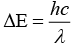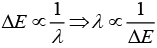As energy separation increases, the wavelength decreases. Thus, the correct order is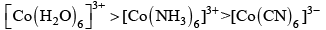Here, strength of ligand increases, AE increases, CFSE increases and X absorbed decreases.
Hence, correct choice is (iii).

Q.3. When 0.1 mol CoCl3(NH3)5 is treated with excess of AgNO3, 0.2 mol of AgCl are obtained. The conductivity of solution will correspond to
(i) 1 : 3 electrolyte
(ii) 1 : 2 electrolyte
(iii) 1 : 1 electrolyte
(iv) 3 : 1 electrolyte
Ans. (ii)
Solution.

One mole of AgNO3 precipitates one mole of chloride ion. In the above reaction, when 0.1 mole of CoCl3(NH3)5 is treated with excess of AgNO3, 0.2 mole of AgCl are obtained thus, there must be two free chloride ions in the solution of electrolyte.
So, molecular formula of complex will be [Co(NH3)5Cl]Cl2 and electrolytic solution must contain [Co(NH3)5Cl]2+ and two Cl- as constituent ions. Thus, it is 1:2 electrolyte.
[Co(NH3)5Cl]CI2 → [Co(NH3)6Cl]2(aq) + 2Cr(aq)
Hence, option (ii) is the correct.

Q.4. When 1 mol CrCl3⋅6H2O is treated with excess of AgNO3, 3 mol of AgCl are obtained. The formula of the complex is :
(i) [CrCl3 (H2O)3]⋅3H2O
(ii) [CrCl2(H2O)4]Cl⋅2H2O
(iii) [CrCl(H2O)5]Cl2⋅H2O
(iv) [Cr(H2O)6]Cl3
Ans.
Solution.

AgNO3 precipitates one free chloride ion (CP).
Here, 3 moles of AgCl are precipitated by excess of AgNO3. Hence, there must be three free Cl- ions.
So, the formula of the complex can be [Cr(H2O)6]Cl3 and correct choice is (iv).

Q.5. The correct IUPAC name of [Pt(NH3)2Cl2] is
(i) Diamminedichloridoplatinum (II)
(ii) Diamminedichloridoplatinum (IV)
(iii) Diamminedichloridoplatinum (0)
(iv) Dichloridodiammineplatinum (IV)
Ans. (i)
Solution.

The complex compound is [Pt(NH3)2Cl2]. The ligands present in the compound are (i) NH3 -neutral ligand represented as amine. (ii) Cl-anion ligand (ending with-o-) represented as chlorido di prefixed to represent two ligands.
The oxidation number of platinum in the compound is 2.Hence, correct IUPAC name of [Pt (NH3)2Cl2] is
Diammine dichloridoplatinum (II)
So, (i) option is correct.

Q.6. The stabilisation of coordination compounds due to chelation is called the chelate effect. Which of the following is the most stable complex species?
(i) [Fe(CO)5]
(ii) [Fe(CN)6]3–
(iii) [Fe(C2O4)3]3–
(iv) [Fe(H2O)6]3+
Ans. (iii)
Solution.

Chelation (formation of cycle by linkage between metal ion and ligand) stabilises the coordination compound. The ligand which chelates the metal ion is known as chelating ligand.
Here, only [Fe(C2O4)3]3− is a coordination compound which contains oxalate ion as a chelating ligand. Hence, it stabilises coordination compound by chelating Fe3+ ion.

Q.7. Indicate the complex ion which shows geometrical isomerism.
(i) [Cr(H2O)4Cl2]+
(ii) [Pt(NH3)3 Cl]
(iii) [Co(NH3)6]3+
(iv) [Co(CN)5(NC)]3–
Ans. (i)
Solution.

[Cr(H2O)4Cl2]shows geometrical isomerism because it is a MA4B2 type coordination compound which contains two sets of equivalent ligands, four H2O and 2Cl.
Hence the possible geometrical isomers are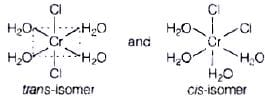Hence, correct choice is (i).

Q.8. The CFSE for octahedral [CoCl6]4– is 18,000 cm–1. The CFSE for tetrahedral [CoCl4]2– will  be
(i) 18,000 cm–1
(ii) 16,000 cm–1
(iii) 8,000 cm–1
(iv) 20,000 cm–1
Ans. (iii)
Solution.

CFSE for octahedral and tetrahedral complexes are closely related to each other by 4 formula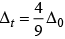where, Δ0 = CFSE for octahedral complex, Δt = CFSE for tetrahedral complex
According to question, Δ0 = 18,000 cm-1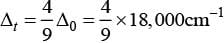= 4 x 2,000 cm-1 = 8,000 cm-1
Hence, correct choice is (iii).

Q.9. Due to the presence of ambidentate ligands coordination compounds show isomerism. Palladium complexes of the type [Pd(C6H5)2(SCN)2] and [Pd(C6H5)2(NCS)2] are
(ii) Coordination isomers
(iii) Ionisation isomers
(iv) Geometrical isomers
Ans. (i)
Solution.

The ligand(s) which has two different bonding sites are known as ambident ligands e.g., NCS,NO2 etc.
Here, NCS has two binding sites at N and S.
Hence, NCS (thiocyanate) can bind to the metal ion in two ways
M ← NCS or M → SNC
Thus, coordination compounds containing NCS as a ligand can show linkage isomerism i.e.,[Pd(C6h5)2(SCN) and [Pd(C6H5)2(NCS)2]are linkage isomers.
Hence, correct choice is (i).

Q.10. The compounds [Co(SO4)(NH3)5]Br and [Co(SO4)(NH3)5]Cl  represent
(ii) Ionisation isomerism
(iii) Coordination isomerism
(iv) No isomerism
Ans. (iv)
Solution.

Compounds having same molecular formula be different structural formula are known as isomers, [Co(SO4)2(NH3)5]Br and [Co(SO4)(NH3)5]Cl molecular formula. Hence, they are not isomers.

Q.11. A chelating agent has two or more than two donor atoms to bind to a single metal ion. Which of the following is not a chelating agent?
(i) Thiosulphato
(ii) Oxalato
(iii) Glycinato
(iv) Ethane-1,2-diamine
Ans. (i)
Solution.

A chelating ligand has two or more-binding donor atoms to a single metal ion.
Thiosulphato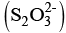has only one donor site, so geometrically it is not favourable for S2O2-3 to chelate a metal ion.

Q.12. Which of the following species is not expected to be a ligand?
(i) NO
(ii)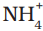(iii) NH2CH2CH2NH2
(iv) CO
Ans. (ii)
Solution.

Ligand must donate a pair of electron or loosely held electron pair to metal and form a M—L bond.
However, among all given speciesdoes not have any pair of electrons, hence it is not a ligand.

Q.13. What kind of isomerism exists between [Cr(H2O)6]Cl3 (violet)  and [Cr(H2O)5Cl]Cl2⋅H2O (greyish-green)?
(ii) Solvate isomerism
(iii) Ionisation isomerism
(iv) Coordination isomerism
Ans. (ii)
Solution.

This form of isomerism is known as hydrate or solvate isomerism in which water is involved as a solvent. This is similar to ionisation isomerism. This isomers differs by whether or not a solvent molecule is directly bonded to the metal ion or merely present as free solvent in the crystal lattice.

Q.14.  IUPAC name of [Pt (NH3)2 Cl(NO2)] is :
(i) Platinum diaminechloronitrite
(ii) Chloronitrito-N-ammineplatinum (II)
(iii) Diamminechloridonitrito-N-platinum (II)
(iv) Diamminechloronitrito-N-platinate (II)
Ans. (iii)
Solution.

Correct IUPAC name can written as
The ligands presents in the given coordination compound are
(i) (NH3) represented as amine
(ii) Cl+ represented as chloriod
(iii) NO2 represented as nitrito-N According to IUPS rule, ligands are named in an alphabetical order before central atom prefix di-will be used to indicate the number of NH3 ligands present.
Oxidation stale of metal is indicated by Roman numeral in parenthesis.
So. IUPAC name will be- diamminenchloronitrito-N-platinum (II)
Hence, option (iii) is correct.

MULTIPLE CHOICE QUESTIONS (TYPE - II)

Note : In the following questions two or more options may be correct.
Q.15. Atomic number of Mn, Fe and Co are 25, 26 and 27 respectively. Which of the following inner orbital octahedral complex ions are diamagnetic?
(i) [Co(NH3)6]3+
(ii) [Mn(CN)6]3–
(iii) [Fe(CN)6]4–
(iv) [Fe(CN)6]3–
Ans. (i, iii)
Solution.

(a) Molecular orbital electronic configuration of Co3+ in [Co(NH2)6]3+ is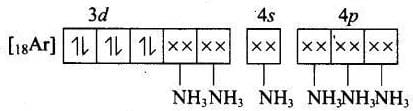Number of unpaired electron = 0
Magnetic property = Diamagnetic
(b) Molecular orbital electronic configuration of Mn3+ in [Mn (CN)6]3-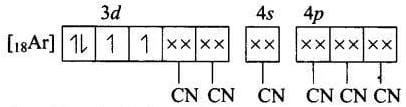Number of unpaired electrons = 2
(c) Magnetic property = Paramagnetic Molecular orbital electronic configuration of Fe2+ in [Fe(CN6]4- is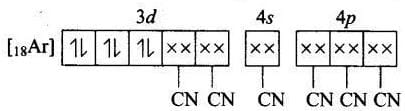Number of unpaired electron = 0
Magnetic property = Diamagnetic
(d) Molecular orbital electronic configuration of Fe3+ in [Fe(CN)6]3-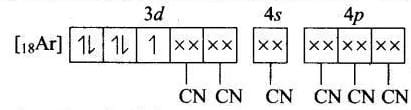Number of unpaired electron = 1
Magnetic property = Paramagnetic
Thus, [Co(NH3)6]3+ and [Fe(CN)6]4- are diamagnetic
Hence, correct choices are options (i) and (iii).

Q.16. Atomic number of Mn, Fe, Co and Ni are 25, 26 27 and 28 respectively. Which of the following outer orbital octahedral complexes have same number of unpaired electrons?
(i) [MnCl6]3–
(ii) [FeF6]3–
(iii) [CoF6]3–
(iv) [Ni(NH3)6]2+
Ans. (i, iii)
Solution.

(a) Molecular orbital electronic configuration of Mn3+ in [MnCl6]3- is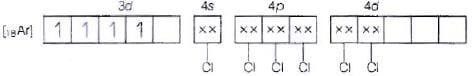Number of unpaired electrons = 4
Magnetic property = Paramagnetic
(b) Molecular orbital electronic configuration of Co3+ in [CoF6]3- is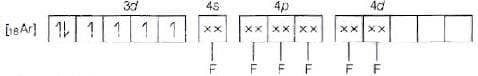Number of unpaired electrons = 4
Magnetic property = Paramagnetic
(c) Molecular orbital electronic configuration of Fe3+ in [FeF6]3- is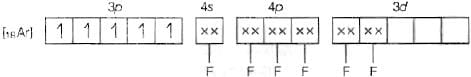Number of unpaired electrons = 5
Magnetic property = Paramagnetic
(d) Molecular orbital electronic configuration of Ni2+ in [Ni(NH3)6]2+ is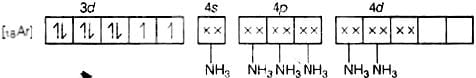Number of unpaired electrons = 5
Magnetic property = Paramagnetic
Thus, [MnCl6]3- and [CoF6]3- are paramagnetic having four electrons each.
Hence, correct choices are (i) and (iii)

Q.17. Which of the following options are correct for [Fe(CN)6]3– complex?
(i) d2sp3 hybridisation
(ii) sp3d2 hybridisation
(iii) Paramagnetic
(iv) Diamagnetic
Ans. (a, c)
Solution.

According to VBT, the molecular orbital electronic configuration of Fe3- in [Fe(CN)6]3- is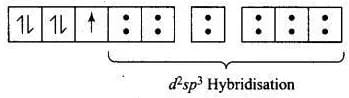Hybridisation = d2sp3
Number of unpaired electron = 1
Magnetic property = Paramagnetic
Hence, correct choices are options (i) and (iii).

Q.18. An aqueous pink solution of cobalt(II) chloride changes to deep blue on addition of excess of HCl. This is because _______.
(i) [Co(H2O)6]2+ is transformed into [CoCl6]4–
(ii) [Co(H2O)6]2+ is transformed into [CoCl4]2–
(iii) Tetrahedral complexes have smaller crystal field splitting than octahedral complexes.
(iv) Tetrahedral complexes have larger crystal field splitting than octahedral complex.
Ans. (ii, iii)
Solution.

Aqueous pink solution of cobalt (II) chloride is due to electronic transition of electron from t2g to eg energy level of [Co(H2O)6]2+ complex. When excess of HCl is added to this solution
(i) [Co(H2O)6f is transformed into [CoCl4]2-
(ii) Tetrahedral complexes have smaller crystal field spiting than octahedral complexes because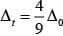Hence, options (ii) and (iii) are correct choices.

Q.19. Which of the following complexes are homoleptic?
(i) [Co(NH3)6]3+
(ii) [Co(NH3)4 Cl2]+
(iii) [Ni(CN)4]2–
(iv) [Ni(NH3)4Cl2]
Ans. (i, iii)
Solution.

Homoleptic complex: The complex containing only one species or group as ligand is known as homoleptic ligand. For example, [Co(NH3)6]3+ [Ni(CN)4]2-
Here, [Co(NH3)6]3+ contain only NH3 as a ligand and [Ni(CN)4]2- contain CN as a ligand.
While other two complexes [Co(NH3)4Cl2]+ and [Ni(NH3)4Cl2] contain NH3 and Cl as ligands.
Hence, options (i) and (iii) are correct choices.

Q.20. Which of the following complexes are heteroleptic?
(i) [Cr(NH3)6]3+
(ii) [Fe(NH3)4 Cl2]+
(iii) [Mn(CN)6]4–
(iv) [Co(NH3)4Cl2]
Ans. (ii, iv)
Solution.

Heteroleptic complexes: Coordination complexes which contain more than one type of ligands are known as heteroleptic complexes.
For example, [Fe(NH3)4CI2]containing NH3 and Cl as ligands is a heteroleptic complex. Similarly, [Co(NH3)4Cl2] containing NH3 and Cl as ligands is also a heteroleptic complex.
Hence, options (ii) and (iv) are correct choices.

Q.21. Identify the optically active compounds from the following :
(i) [Co(en)3]3+
(ii) trans– [Co(en)2 Cl2]+
(iii) cis– [Co(en)2 Cl2]+
(iv) [Cr (NH3)5Cl]

Ans. (i, iii)
Solution.

[Co(en)3]3+ and cis-[Co(en)2Cl2]+ are optically active compounds because their mirror images are non-superimposable isomer.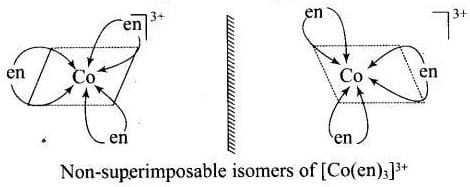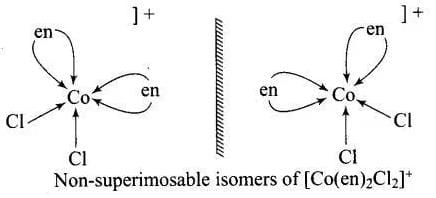Hence, (i) and (iii) are correct choices.

Q.22. Identify the correct statements for the behaviour of ethane-1, 2-diamine as a ligand.
(i) It is a neutral ligand.
(ii) It is a didentate ligand.
(iii) It is a chelating ligand.
(iv) It is a unidentate ligand.

Ans. (i, ii, iii)
Solution.

Molecular formula of ethane-1, 2-diamine is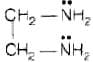(i) Ethane-1, 2-diamine is a neutral ligand due to absence of any charge
(ii) It is a didentate ligand due to presence of two donor sites one at each nitrogen atom of amino group.
(iii) It is a chelating, ligand due to its ability to chelate with the metal.
Hence, options (i), (ii) and (iii) are correct choices.

Q.23. Which of the following complexes show linkage isomerism?
(i) [Co(NH3)5 (NO2)]2+
(ii) [Co(H2O)5CO]3+
(iii) [Cr(NH3)5 SCN]2+
(iv) [Fe(en)2 Cl2]+
Ans. (i,iii)
Solution.

Coordination compounds containing a ligand with more than one non-equivalent binding position (known as ambident ligand) show linkage isomerism.
e.g., [Co(NH3)5(NO2)+ contains NO2 which have two donor sites N and O can be shown by arrow (→) as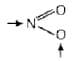[Cr(NH3)5SCN]s+ contains SCN which have two different donor sites S and N can be shown by arrow (→) as
→S - C ≡ N ←
Hence, [Co(NH3)s(NO2)]2+ and [Cr(NH3):SCN]2+ show linkage isomerism. While [Co(H2O)5 CO]3+ and [Fe(en)2Cl2]+ has no ambident ligand, So, these two will not show linkage isomerism.
Hence, options (i) and (iii) are correct choices

Q.24. Arrange the following  complexes in the increasing order of conductivity of their solution: [Co(NH3)3Cl3], [Co(NH3)4Cl2]Cl, [Co(NH3)6]Cl3 , [Cr(NH3)5Cl]Cl2
Ans. Ions or molecules present outside the coordination Sphere are ionisable A complex which gives more ions on dissolution, is more conducting.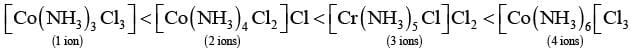Here, number of ions increases and conductivity increases

Q.25. A coordination compound CrCl3⋅4H2O precipitates silver chloride when treated with silver nitrate. The molar conductance of its solution corresponds to a total of two ions. Write structural formula of the compound and name it.
Ans. Formation of white precipitate with AgNO3 shows that at least one Cl- ion is present outside the coordination sphere. Moreover only two ions are obtained in solution, so only one Cl- present outside the sphere.  Thus, the formula of the complex is [Co(H2O)4Cl2]Cl and its IUPAC name is Tetraaquadichloridocobalt (III) chloride.

Q.26. A complex of the type [M(AA)2X2]n+ is known to be optically active. What does this indicate about the structure of the complex? Give one example of such complex.
Ans. An optically active complex of the type [M(AA)2X2]n+ indicates cis-octahedral structure,
e.g., cis-[Pt (en)2Cl2]2+ or cis-[Cr(en)2Cl2]because its mirror image isomers are non superimposable.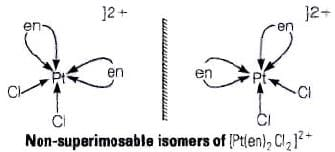Non-superimposable isomers of [Pt(en)2CI2]2+.

Q.27. Magnetic moment of [MnCl4]2– is 5.92 BM. Explain giving reason.
Ans. The magnetic moment 5.92 BM shows that there are five unpaired electrons present in the d-orbitals of Mn2+ ion. As a result, the hybridisation involved is sp3 rather than dsp2. Thus tetrahedral structure of [MnCl4]2− complex will show 5.92 BM magnetic moment value.

Q.28. On the basis of crystal field theory explain why Co(III) forms paramagnetic octahedral complex with weak field ligands whereas it forms diamagnetic octahedral complex with strong field ligands.
Ans. With weak field ligands; ∆0 < P, (pairing energy) so, the electronic configuration of Co (III) will be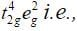it has 4 unpaired electrons and is paramagnetic.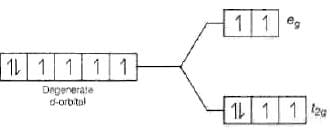With strong field ligands, ∆0 < P, (pairing energy), so pairing occurs thus, the electronic configuration will be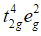It has no impaired electrons and is diamagnetic.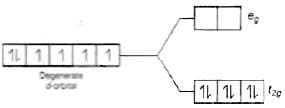Q.29. Why are low spin tetrahedral complexes not formed?

Ans. In tetrahedral complex, the d-orbital is splitting to small as compared to octahedral. For same metal and same ligand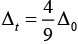Hence the orbital splitting energies are not enough to force pairing. As a result, low spin configurations are rarely observed in tetrahedral complexes.

Q.30. Give the electronic configuration of the following complexes on the basis of crystal field splitting theory.

[CoF6]3–, [Fe(CN)6]4– and [Cu(NH3)6]2+.
Ans. According to spectrochemical series, ligands can be arranged in a series in the order of increasing field strength i.e., F- < NH3 <CN-
Hence, CN- and NH3 being strong field ligand pair up the t2g electrons before filling egset.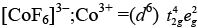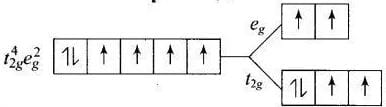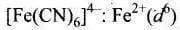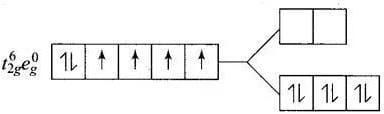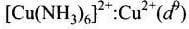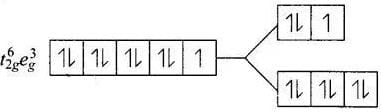Q.31. Explain why [Fe(H2O)6]3+ has magnetic moment value of 5.92 BM whereas [Fe(CN)6]3– has a value of only 1.74 BM.
Ans. As we know,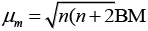where,  μm= magnetic moment
μn. = number of unpaired electrons
Here, μn= 1.74 i.e., n = 1
and μ= 5.92 i.e., n = 5
[Fe(CN)6]3- involves d2sp3 hybridisation with one unpaired electron (magnetic moment 1.74 BM) and [Fe(H2O)6]3+ involves sp3d2 hybridisation with five unpaired electrons (because magnetic moment equal to 5.92 BM).
CN- is stronger ligand than H2O according to spectrochemical series. ∆0 > P for CN- fourth electron will pair itself. Whereas for water pairing will not happen for [Fe(CN)6]3- electronic configuration of Fe3+ is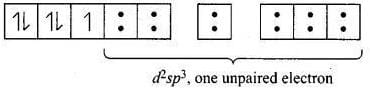One unpaired electron
For [Fe (H2O) 6]3+ the electronic configuration of Fe3+ is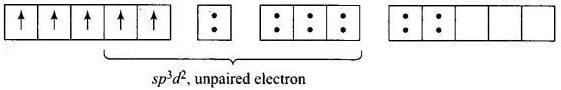Five unpaired electron Hence, [Fe(CN)6]3- and [Fe(H2O)6f: are inner orbital and outer orbital complex respectively.

Q.32. Arrange following complex ions in increasing order of crystal field splitting energy (∆O) :

[Cr(Cl)6]3–, [Cr(CN)6]3–, [Cr(NH3)6]3+.
Ans. CFSE is higher when the complex contains strong field ligand. Thus, crystal field splitting energy increases in the order
[Cr(Cl)6]3- < Cr(CN)6]3+< [Cr(NH3)6]3+
Because according to spectrochemical series the order of field strength is
Cl< NH3 < CN-

Q.33. Why do compounds having similar geometry have different magnetic moment?
Ans. It is due to the presence of weak and strong field ligands in complexes. If CFSE is high, the complex will show low value of magnetic moment and vice-versa, e.g. [CoF6]3- and [Co(NH3)6]3-, the formal is paramagnetic, and the latter is diamagnetic because F- is a weak field ligand and NIH3 is a strong field ligand while both have similar geometry.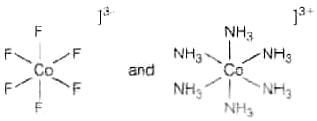Q.34. CuSO4.5H2O is blue in colour while CuSO4 is colourless. Why?

Ans. In CuSO4.5H2O, water acts as ligand and causes crystal field splitting. Hence, d-d transition is possible thus CuSO4. H2O is coloured. On the other hand, in the anhydrous CuSO4 due to the absence of water (ligand), crystal field splitting is not possible and hence, it is colourless.

Q.35. Name the type of isomerism when ambidentate ligands are attached to central metal ion. Give two examples of ambidentate ligands.

Ans. Ligands having more than one different binding positions are known as ambidentate ligands. e.g., SCN has two different binding positions S and N. Coordination compound containing ambidentate ligands are considered to show linkage isomerism due to presence of two different binding positions.
e.g., (i) [Co(NH3)5SCN]3+ and
(ii) [Fe(NH3)5(NO2)]3+

MATCHING TYPE

Note : In the following questions match the items given in Columns I and II.
Q.36. Match the complex ions given in Column I with the colours given in Column II and assign the correct code :

 Column I (Complex ion) Column II (Colour) A. [Co(NH3)6]3+ 1. Violet B. [Ti(H2O)6]3+ 2. Green C. [Ni(H2O)6]2+ 3. Pale blue D. (Ni (H2O)4 (en)]2+ (aq) 4. Yellowish orange 5. Blue

Code :
(i) A → (1) B → (2) C → (4) D → (5)
(ii) A → (4) B → (3) C → (2) D → (1)
(iii) A → (3) B → (2) C → (4) D → (1)
(iv) A → (4) B → (1) C → (2) D → (3)

Ans. (iv)
Solution.
Colour of coordination compound is closely related to CFSE to coordination compound. Depending upon the CFSE of given coordination compounds Correct matching will be as follows

 Column I (Complex ion) Column II (Colour) A. [Co(NH3)6]3+ 4. Yellowish orange B. [Ti(H2O)6]3+ 1. Violet C. [Ni(H2O)6]2+ 2. Green D. (Ni (H2O)4 (en)]2+ (aq) 3. Pale blue

Hence, correct choice is (iv).

Q.37. Match the coordination compounds given in Column I with the central metal atoms given in Column II and assign the correct code :

 Column I (Coordination Compound) Column II (Central metal atom) A. Chlorophyll 1. rhodium B. Blood pigment 2. cobalt C. Wilkinson catalyst 3. magnesium D. Vitamin B12 4. iron 5. calcium

Code :
(i) A → (5) B → (4) C → (1) D → (2)
(ii) A → (3) B → (4) C → (5) D → (1)
(iii) A → (4) B → (3) C → (2) D → (1)
(iv) A → (3) B → (4) C → (1) D → (2)

Ans. (iv)
Solution.

Central metal ions present on coordination compounds determine the properties of coordination compound and their biological role.

 Column I Column II A. Chlorophyll 3. calcium B. Blood pigment 4. iron C. Wilkinson catalyst 1. rhodium D. Vitamin B12 2. cobalt

Hence, correct choice is (iv).

Q.38. Match the complex ions given in Column I with the hybridisation and number of unpaired electrons given in Column II and assign the correct code :

 Column I (Complex ion) Column II (Hybridisation, number of unpaired electrons) A. [Cr(H2O)6]3+ 1.  dsp 2, 1 B. [Co(CN)4]2– 2.  sp3d2, 5 C. [Ni(NH3)6]2+ 3.  d2sp3, 3 D. [MnF6]4– 4.  sp3, 4 5.  sp3d2, 2

Code :
(i) A → (3) B → (1) C → (5) D → (2)
(ii) A → (4) B → (3) C → (2) D → (1)
(iii) A → (3) B → (2) C → (4) D → (1)
(iv) A → (4) B → (1) C → (2) D → (3)

Ans.  (i)
Solution.

Formation of inner orbital complex and outer orbital complex determines hybridisation of molecule which inturn depends upon field strength of ligand and number of vacant d orbitals.
(i) Strong field ligand forms inner orbital complex with hybridisation d2sp3.
(ii) Weak field ligand forms outer orbital complex with hybridisation sp3d2.
According to VBT, hybridisation and number of unpaired electrons of coordination compounas can be calculated as
(a) [Cr(H2O)]3+
MOEC (Molecular orbital electronic configuration) of Cr3+ in [Cr(H2O)8]3+ is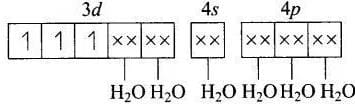Hybridisation = d2sp3
n (number of unpaired electrons) = 3
(b) [Co(CN)4]2−
MOEC of Co2+ in [Co(CN)4]2− is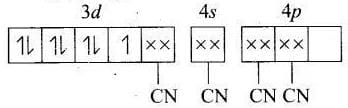Hybridisation =dsp2
n = 1
(c) [Ni(NH3)6]2−
MOEC of Ni2+ in [Ni(Nh3)6]2+ is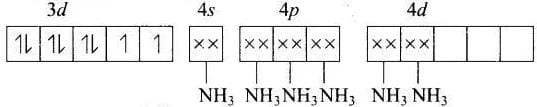Hybridisation = sp3d2
n = 2
(d) [MnF6]4−
MOEC of Mn2+ in [MnF6]4− is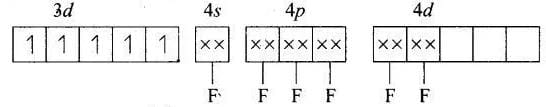Hybridisation = sp3d2
n = 5
Hence, correct choice can be represented by (i).

Q.39. Match the complex species given in Column I with the possible isomerism given in Column II and assign the correct code :

 Column I (Complex species) Column II (Isomerism) A. [Co(NH3)4 Cl2]+ 1. optical B. cis-[Co(en)2 Cl2]+ 2. ionisation C. [Co(NH3)5 (NO2)]Cl2 3. coordination D. [Co(NH3)6][Cr(CN)6] 4. geometrical 5. linkage

Code :
(i) A → (1) B → (2) C → (4) D → (5)
(ii) A → (4) B → (3) C → (2) D → (1)
(iii) A → (4) B → (1) C → (5) D → (3)
(iv) A → (4) B → (1) C → (2) D → (3)

Ans. (iv)
Solution.

Isomerism in coordination compound is decided by type of ligands and geometry of coordination and arrangement of ligands.
(a) [Co(NH3)4Cl2]+ shows geometrical isomerism due to presence of two types of ligand whose [Co(NH3)4 Cl2]+ arrangement around central metal ion.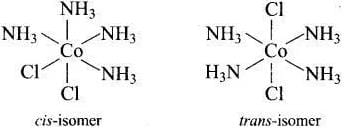(b) cis-[Co(en)2Cl2]+ shows optical isomer due to its non-superimposable mirror image relations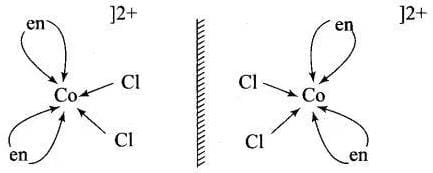(c) [Co(NH3)5(NO2)]Clshows ionisation isomer due to its interchanging ligand from outside the ionisation sphere.
(d) [Co(NH3)6][Cr(CN)6]shows coordination isomer due to interchanging of ligand in between two metal ions from one coordination sphere to another coordination sphere.
Hence,correct choice is (iv).

Q.40. Match the compounds given in Column I with the oxidation state of cobalt present in it (given in Column II) and assign the correct code.

 Column I (Compound) Column II (Oxidation state of Co) A. [Co(NCS)(NH3)5](SO3) 1. +4 B. [Co(NH3)4Cl2]SO4 2. 0 C. Na4[Co(S2O3)3] 3. +1 D. [Co2(CO)8] 4. +2 5. +3

Code :
(i) A → (1) B → (2) C → (4) D → (5)
(ii) A → (4) B → (3) C → (2) D → (1)
(iii) A → (5) B → (1) C → (4) D → (2)
(iv) A → (4) B → (1) C → (2) D → (3)

Ans. (iii)
Solution.

The correct answer will be the option (iii) A → (5) B → (1) C → (4) D → (2)
Hence, matching the two columns the right combination will be:
A. [Co(NCS)(NH3)5](SO3) -5. + 3 (oxidation no.of Co, x =-1(-1)-(-2)=+3)
B. [Co(NH3)4Cl2]SO4 -1. + 4 (oxidation no. Of Co, x =-2x(-2)-1(-2)=+4)
C. Na4[Co(S2O3)3] -4.+ 2 (oxidation no. Of Co, x=-3(-2) – 4x(+1)=+2 )
D. [Co2(CO)8] –2. 0. (as CO is a neutral ligand, therefore Co here has a zero oxidation state)

ASSERTION AND REASON TYPE

Note : In the following questions a statement of assertion followed by a statement of reason is given. Choose the correct answer out of the following choices.
(i) Assertion and reason both are true, reason is correct explanation of assertion.
(ii) Assertion and reason both are true but reason is not the correct explanation of assertion.
(iii) Assertion is true, reason is false.
(iv) Assertion is false, reason is true.

Q.41. Assertion : Toxic metal ions are removed by the chelating ligands.
Reason : Chelate complexes tend to be more stable.
(i) Assertion and reason both are true, reason is correct explanation of assertion.
(ii) Assertion and reason both are true but reason is not the correct explanation of assertion.
(iii) Assertion is true, reason is false.
(iv) Assertion is false, reason is true.

Ans. (i)
Solution.
Toxic metal ions are removed by chelating ligands. When a solution of chelating ligand is added to solution containing toxic metals, ligands chelate the metal ions by formation of stable complex.

Q.42. Assertion : [Cr(H2O)6]Cl2 and [Fe(H2O)6]Clare reducing in nature.
Reason : Unpaired electrons are present in their d-orbitals.
(i) Assertion and reason both are true, reason is correct explanation of assertion.
(ii) Assertion and reason both are true but reason is not the correct explanation of assertion.
(iii) Assertion is true, reason is false.
(iv) Assertion is false, reason is true.

Ans. (ii)
Solution.
Correct reason is [Cr(H2O6)]Cl2 and [Fe(H2O)6]Cl2 are reducing in nature due to formation of more stable complex ion after gaining of electron.

Q.43. Assertion : Linkage isomerism arises in coordination compounds containing ambidentate ligand.
Reason : Ambidentate ligand has two different donor atoms.
(i) Assertion and reason both are true, reason is correct explanation of assertion.
(ii) Assertion and reason both are true but reason is not the correct explanation of assertion.
(iii) Assertion is true, reason is false.
(iv) Assertion is false, reason is true.

Ans. (i)
Solution.
Linkage isomerism arises in coordination compounds containing ambidentate ligands because ambidentate ligand has two different donor atoms. e.g., SCN, NO2 etc.

Q.44. Assertion : Complexes of MXand MX5L type (X and L are unidentate) do not show geometrical isomerism.
Reason : Geometrical isomerism is not shown by complexes of coordination number 6.
(i) Assertion and reason both are true, reason is correct explanation of assertion.
(ii) Assertion and reason both are true but reason is not the correct explanation of assertion.
(iii) Assertion is true, reason is false.
(iv) Assertion is false, reason is true.

Ans. (ii)
Solution.
Complexes of MX6 and MX5L type (X and L are unidentate) do not show geometrical isomerism due to presence of plane of symmetry and necessary condition for showing geometrical isomerism is that complex is must of MA4B2 type or [M(AB)2X2] type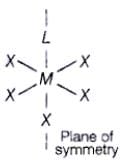Q.45. Assertion : ([Fe(CN)6]3– ion shows magnetic moment corresponding to two unpaired electrons.
Reason : Because it has d2sp3 type hybridisation.
(i) Assertion and reason both are true, reason is correct explanation of assertion.
(ii) Assertion and reason both are true but reason is not the correct explanation of assertion.
(iii) Assertion is true, reason is false.
(iv) Assertion is false, reason is true.

Ans. (iv)
Solution.
According to VBT, MOEC of Fe3+ in [Fe(CN)6]3+ is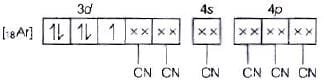Hybridisation =d2sp3
n = 1
Hence, correct assertion is
[Fe(CN)6]3− ion shows magnetic moment corresponding to one unpaired electron.
i.e.,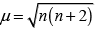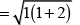Q.46. Using crystal field theory, draw energy level diagram, write electronic configuration of the central metal atom/ion and determine the magnetic moment value in the following :
(i) [CoF6]3–, [Co(H2O)6]2+ , [Co(CN)6]3–
(ii) [FeF6]3–, [Fe(H2O)6]2+, [Fe(CN)6]4–
Ans. (a) [CoF6]3−
F is a weak field ligand.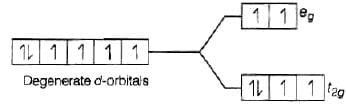Configuration of Co3+ =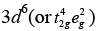Number of unpaired electrons (n) = 4
Magnetic moment (μ) =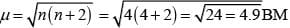[Co(H2O)6]2+,
H2O is a weak field ligand.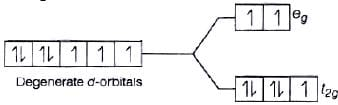Configuration of Co2+ = 3d7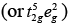Number of unpaired electrons (n) = 3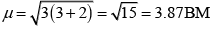[Co(CN)6]3− i.e., Co3+
∵ CN is strong field ligand.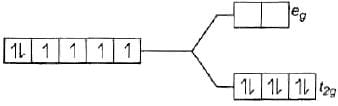Co3+ =3d6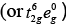There is no unpaired electron, so it is diamagnetic, i.e., μ = 0
(b) [FeF6]3−,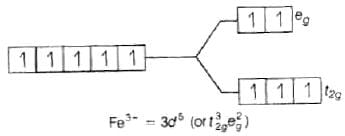Number of unpaired electrons, n = 5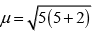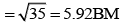[Fe(H2O)6]2+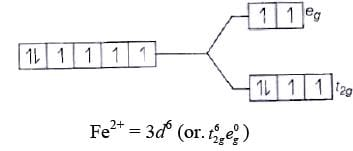Number of unpaired electrons, n = 4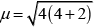Z = √24
= 4.98 BM
[Fe(CN)6]4−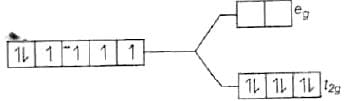Since, CN is a strong field ligand, all the electrons get paired.
Fe2+ = 3d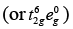Because there is no unpaired electron, so it is diamagnetic in nature, i.e., μ = 0

Q.47. Using valence bond theory, explain the following in relation to the complexes given below: [Mn(CN)6]3– , [Co(NH3)6]3+, [Cr(H2O)6]3+ ,  [FeCl6]4–
(i) Type of hybridisation.
(ii) Inner or outer orbital complex.
(iii) Magnetic behaviour.
(iv) Spin only magnetic moment value.
Ans. (a) [Mn(CN)6]3−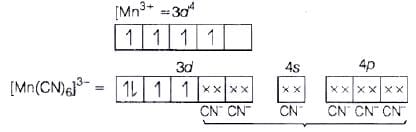(i) d2sp3 hybridisation
(ii) Inner orbital complex because (n −1) d-orbitals are used.
(iii) Paramagnetic, as two unpaired electrons are present.
(iv) Spin only magnetic moment (μ) =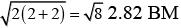(b) [Co(NH3)6]3+
Co3+ =3d6 4s0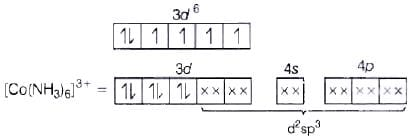(NH3 pair up the unpaired 3d electrons.)
(i) d2sp3 hybridisation
(ii) Inner orbital complex because of the involvement of (n −1) d-orbital in bonding.
(iii) Diamagnetic, as no unpaired electron is present.
(iv)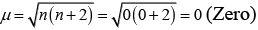(c) [Cr(H20)6]3+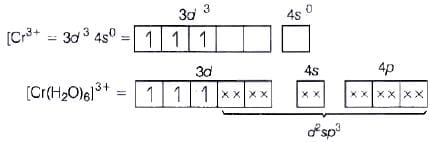(i) d2sp3 hybridisation
(ii) Inner orbital complex (as (n −1) d-orbital take part.)
(iii) Paramagnetic (as three unpaired electrons are present.)
(iv)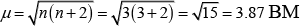(d) [Fe(Cl)6]4−
Fe2+ = 3d6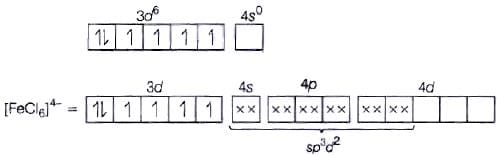(i) sp3d2 hybridisation
(ii) Outer orbital complex because nd-orbitals are involved in hybridisation.
(iii) Paramagnetic (because of the presence of four unpaired electrons).
(iv)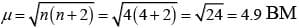Q.48. CoSO4Cl.5NH3 exists in two isomeric forms ‘A’ and ‘B’. Isomer ‘A’ reacts with AgNO3 to give white precipitate, but does not react with BaCl2. Isomer ‘B’ gives white precipitate with BaCl2 but does not react with AgNO3. Answer the following questions.
(i) Identify ‘A’ and ‘B’ and write their structural formulas.
(ii) Name the type of isomerism involved.
(iii) Give the IUPAC name of ‘A’ and ‘B’.

Ans. 'A' gives precipitate with AgNO3, so in it Cl is present outside the coordination sphere.
'B' gives precipitate with BaCl2, so in it SO2-4 is present outside the coordination sphere. (a) So, A-[Co(NH3)5 SO4] Cl
B-[Co(NH3)5 Cl]SO4
(b)Ionisation isomerism (as give different ions when subjected to ionisation.)
(c) [A] Pentaamminesulphatocobalt (III) chloride.
(d)[B], Pentaamminechloridocobalt (III) sulphate.

Q.49. What is the relationship between observed colour of the complex and the wavelength of light absorbed by the complex?
Ans. When white light falls on the complex, some part of it is absorbed. Higher the crystal field splitting energy, lower will be the wavelength absorbed by the complex. The observed colour of complex is the colour generated from the wavelength left over.
e.g., if green light is absorbed, the complex appears red.
In terms of crystal field theory, suppose there is an octahedral complex with empty eg level and unpaired electrons in ther2g level in ground level. If the unpaired electron absorbs light corresponding is blue-green region, it will excite to eg level and the complex will appear violet in colour.
In absence of ligand, crystal field splitting does not occur and the substance is colourless e.g., anhydrous CuSO4 is while, butCuSO4.5H2O is blue in colour.

Q.50. Why are different colours observed in octahedral and tetrahedral complexes for the same metal and same ligands?
Ans. Extent of splitting of d-orbitals is different in octahedral and tetrahedral field. CFSE in octahedral and tetra federal field are closely related as.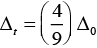where, Δ0 = crystal field splitting energy in tetrahedral field
Δ0 = crystal field splitting energy in octahedral field
Wavelength of light and CFSE are related to each other by formula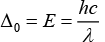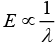So, higher wavelength of light is absorbed in octahedral complexes than tetrahedral complexes for same metal and ligands. Thus, different colours are observed.

The document NCERT Exemplar: Coordination Compounds | Chemistry Class 12 - NEET is a part of the NEET Course Chemistry Class 12.
All you need of NEET at this link: NEET

## FAQs on NCERT Exemplar: Coordination Compounds - Chemistry Class 12 - NEET

 1. What are coordination compounds?Ans. Coordination compounds are complex molecules or ions in which a central metal atom or ion is surrounded by a group of bonded molecules or ions, known as ligands. These ligands donate a pair of electrons to the metal atom/ion, forming coordinate bonds.
 2. How are coordination compounds named?Ans. Coordination compounds are named by writing the name of the ligands first, in alphabetical order, followed by the name of the central metal atom/ion. The oxidation state of the metal is also indicated by using Roman numerals in parentheses, if necessary.
 3. What is the importance of coordination compounds?Ans. Coordination compounds have several important applications. They are widely used as catalysts in chemical reactions, as dyes and pigments, in medicine for the treatment of diseases, and in the extraction of metals from ores. They also play a crucial role in biological processes such as respiration and photosynthesis.
 4. How do coordination compounds form coordination bonds?Ans. In coordination compounds, the ligands donate a pair of electrons to the central metal atom/ion to form coordination bonds. This donation of electrons occurs due to the presence of lone pairs of electrons on the ligands, which are attracted to the positively charged metal atom/ion.
 5. What is the difference between coordination compounds and complex ions?Ans. Coordination compounds refer to the overall complex molecule or ion in which the central metal atom/ion is surrounded by ligands. Complex ions, on the other hand, refer specifically to the charged species formed when a coordination compound is dissolved in a solvent. Complex ions have an overall charge due to the presence of excess positive or negative charges on the ligands or metal atom/ion.

## Chemistry Class 12

154 videos|396 docs|261 tests

## Chemistry Class 12

154 videos|396 docs|261 tests

### How to Prepare for NEET

Read our guide to prepare for NEET which is created by Toppers & the best Teachers
Signup to see your scores go up within 7 days! Learn & Practice with 1000+ FREE Notes, Videos & Tests.
10M+ students study on EduRev
Track your progress, build streaks, highlight & save important lessons and more!(Scan QR code)
Related Searches

,

,

,

,

,

,

,

,

,

,

,

,

,

,

,

,

,

,

,

,

,

;